# High School Chemistry : Identifying Periodic Trends

## Example Questions

← Previous 1 3

### Example Question #1 : The Periodic Table

Which of the following atoms is the largest?

Nitrogen

Lithium

Bromine

Oxygen

Potassium

Potassium

Explanation:

Atom size is determined by the number of shells and how many protons and electrons are present in the atom. Any atom in a higher number period (row) is larger than any atom in a lower number period, since the number of electron shells increases as the number of the period increases. Within the same period, atoms get smaller as you move towards the right. This is because the atomic number increases, increasing the number of protons in the nucleus. These protons attract the surrounding electrons, pulling them in closer, and shrinking the total size of the atom.

In general, atomic radius will increase as you move down and to the left. This makes helium the smallest atom and francium the largest.

### Example Question #2 : The Periodic Table

Which ion of nitrogen is the largest?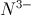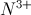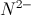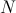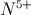Explanation:

Ions of atoms vary in their size. When an atom gains electrons the atomic size increases, and when an atom loses electrons the atomic size decreases. Nitrogen with a formal charge of negative three will have the largest size because it has the most electrons. Remember that adding electrons will cause a negative ion, while removing electrons will cause a positive ion.

### Example Question #3 : The Periodic Table

Which of the following values will decrease as you move left to right in a period of the periodic table?

Atomic number

Electronegativity

Electron affinity

Explanation:

Moving left to right on the periodic table causes an increase in atomic number (number of protons) as well as electron affinity and electronegativity.

Atomic radius, however, will decrease when moving left to right. As more protons are added to the nucleus, they have a stronger attraction to the electrons. This pulls the electrons closer to the nucleus, resulting in a smaller atomic radius.

### Example Question #1 : The Periodic Table

Elements that have similar properties will have the same __________ on the periodic table.

group

period

atomic mass

atomic charge

group

Explanation:

The periodic table is set up so that we can quickly tell which elements will react in a similar chemical fashion. The elements from the same group, that is the same column, will have similar properties. This is due to the same number of valence electrons in the outer shell.

### Example Question #2 : Identifying Periodic Trends

Elements from which group in the periodic table will have the lowest first ionization energies?

Halogens

Alkali metals

Group III

Alkaline earth metals

Noble gases

Alkali metals

Explanation:

Ionization energy is the amount of energy needed to remove an electron from an atom. Alkali metals only need to lose one electron in order to achieve the stable noble gas octet. As a result, the loss of this electron requires very little energy since the resulting ion is favorably stable. This property causes alkali metals (found in group I) to have the lowest first ionization energies.

### Example Question #1 : The Periodic Table

Which of these elements has the smallest atomic radius?

Phosphorus

Bismuth

Arsenic

Antimony

Nitrogen

Nitrogen

Explanation:

The trend for atomic radius is as follows: atomic radius increases from right to left within a row and from top to bottom within a group on the periodic table.

### Example Question #1 : The Periodic Table

Which of these elements is the most electronegative?

Carbon

Boron

Oxygen

Nitrogen

Fluorine

Fluorine

Explanation:

Fluorine is the most electronegative element. The trend for electronegativity is as follows: electronegativity increases from left to right within a row and from bottom to top within a group of the periodic table.

### Example Question #1 : The Periodic Table

Which of these elements is the least electronegative?

Potassium

Francium

Cesium

Rubidium

Sodium

Francium

Explanation:

Francium is the least electronegative element. The trend for electronegativity is as follows: electronegativity increases from left to right within a row and from bottom to top within a group of the periodic table.

### Example Question #1 : The Periodic Table

Which of these elements has the largest atomic radius?

Sulfur

Chlorine

Silicon

Phosphorus

Aluminum

Aluminum

Explanation:

The trend for atomic radius is as follows: atomic radius increases from right to left within a row and from top to bottom within a group on the periodic table.

### Example Question #1 : Identifying Periodic Trends

Which of these elements has the greatest ionization energy?

Cesium

Sodium

Lithium

Potassium

Rubidium